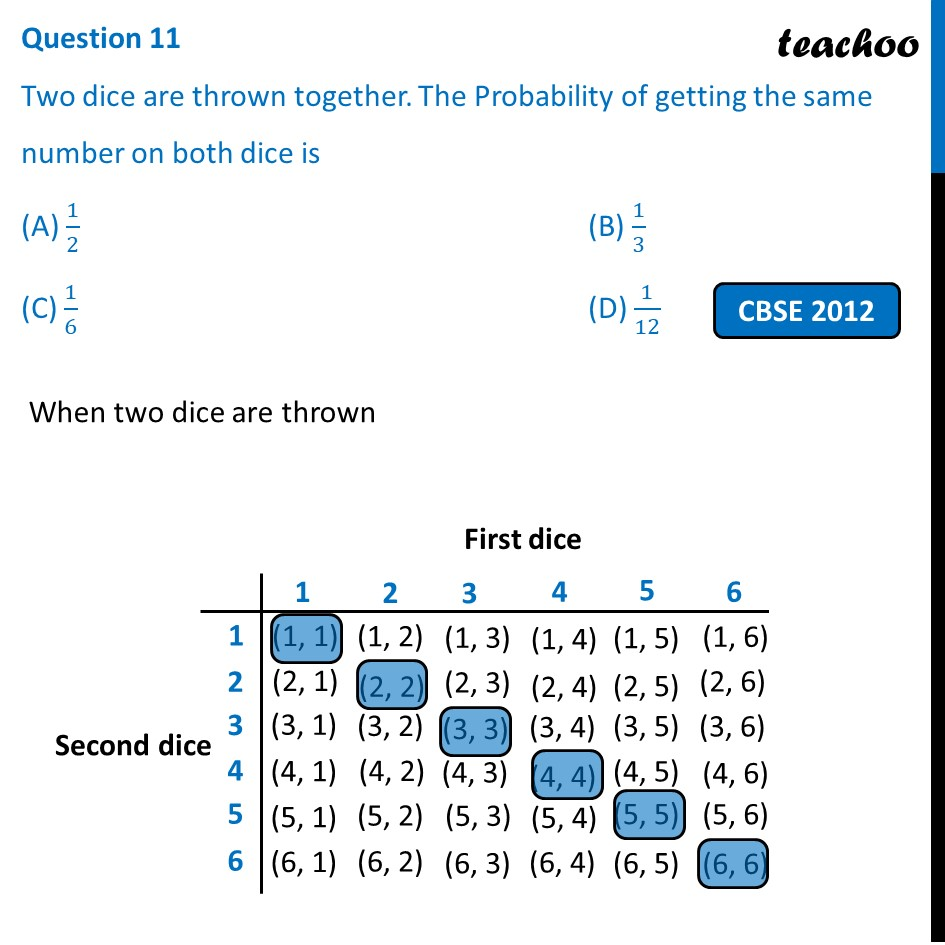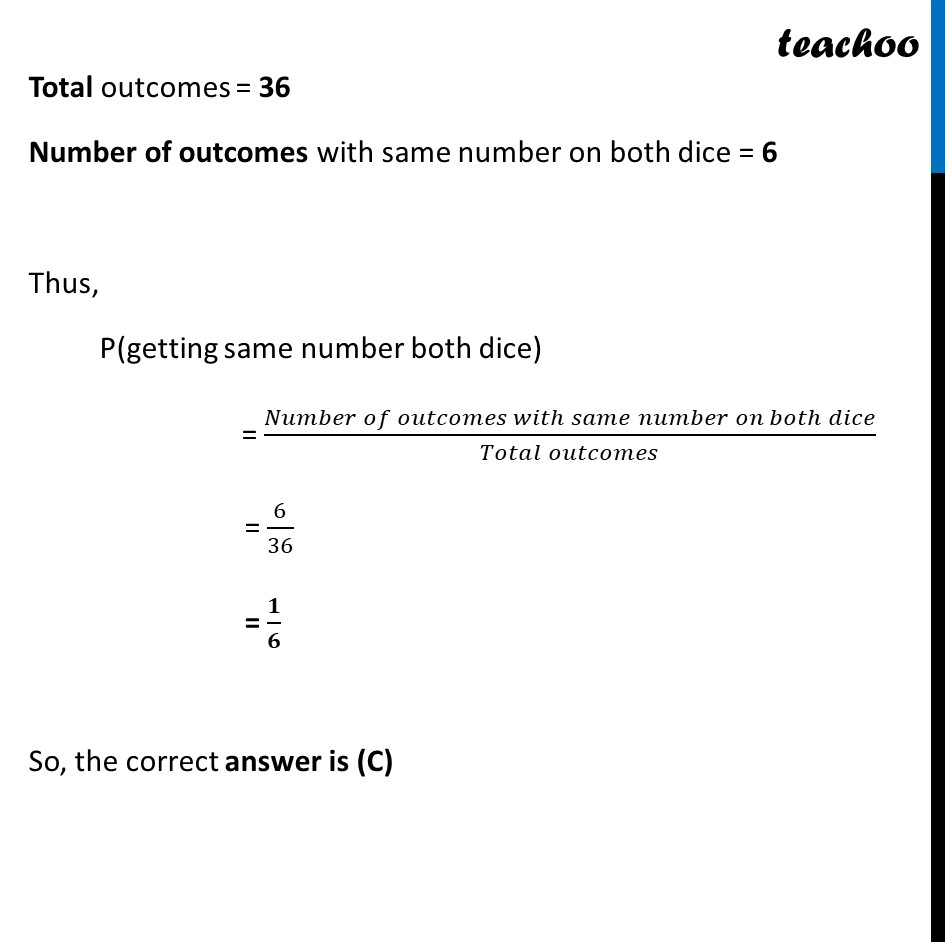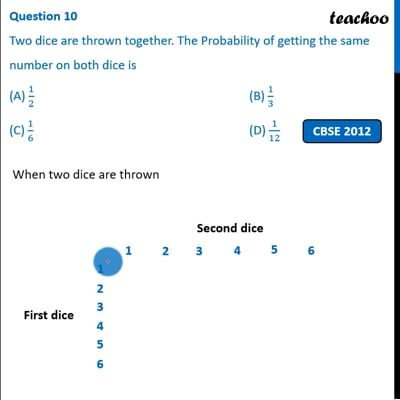Past Year MCQ

Chapter 15 Class 10 Probability (Term 1)
Serial order wise

## (D) 1/12

This question is simlar to Question 8 - Past Year MCQ - Chapter 15 Class 10 ProbabilityThis video is only available for Teachoo black users

### Transcript

Question 11 Two dice are thrown together. The Probability of getting the same number on both dice is (A) 1/2 (B) 1/3 (C) 1/6 (D) 1/12 When two dice are thrown Total outcomes = 36 Number of outcomes with same number on both dice = 6 Thus, P(getting same number both dice) = (𝑁𝑢𝑚𝑏𝑒𝑟 𝑜𝑓 𝑜𝑢𝑡𝑐𝑜𝑚𝑒𝑠 𝑤𝑖𝑡ℎ 𝑠𝑎𝑚𝑒 𝑛𝑢𝑚𝑏𝑒𝑟 𝑜𝑛 𝑏𝑜𝑡ℎ 𝑑𝑖𝑐𝑒)/(𝑇𝑜𝑡𝑎𝑙 𝑜𝑢𝑡𝑐𝑜𝑚𝑒𝑠) = 6/36 = 𝟏/𝟔 So, the correct answer is (C)